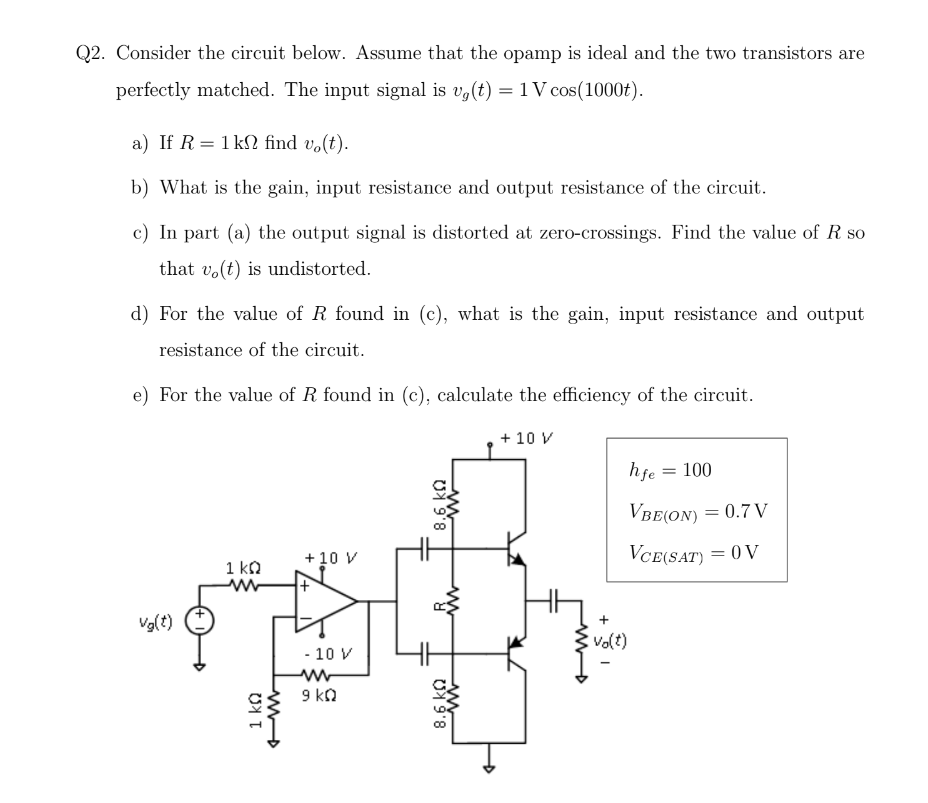Home / Expert Answers / Electrical Engineering / 2-consider-the-circuit-below-assume-that-the-opamp-is-ideal-and-the-two-transistors-are-perfectly-pa530

# (Solved): 2. Consider the circuit below. Assume that the opamp is ideal and the two transistors are perfectly ...2. Consider the circuit below. Assume that the opamp is ideal and the two transistors are perfectly matched. The input signal is . a) If find . b) What is the gain, input resistance and output resistance of the circuit. c) In part (a) the output signal is distorted at zero-crossings. Find the value of so that is undistorted. d) For the value of found in (c), what is the gain, input resistance and output resistance of the circuit. e) For the value of found in (c), calculate the efficiency of the circuit.

We have an Answer from Expert